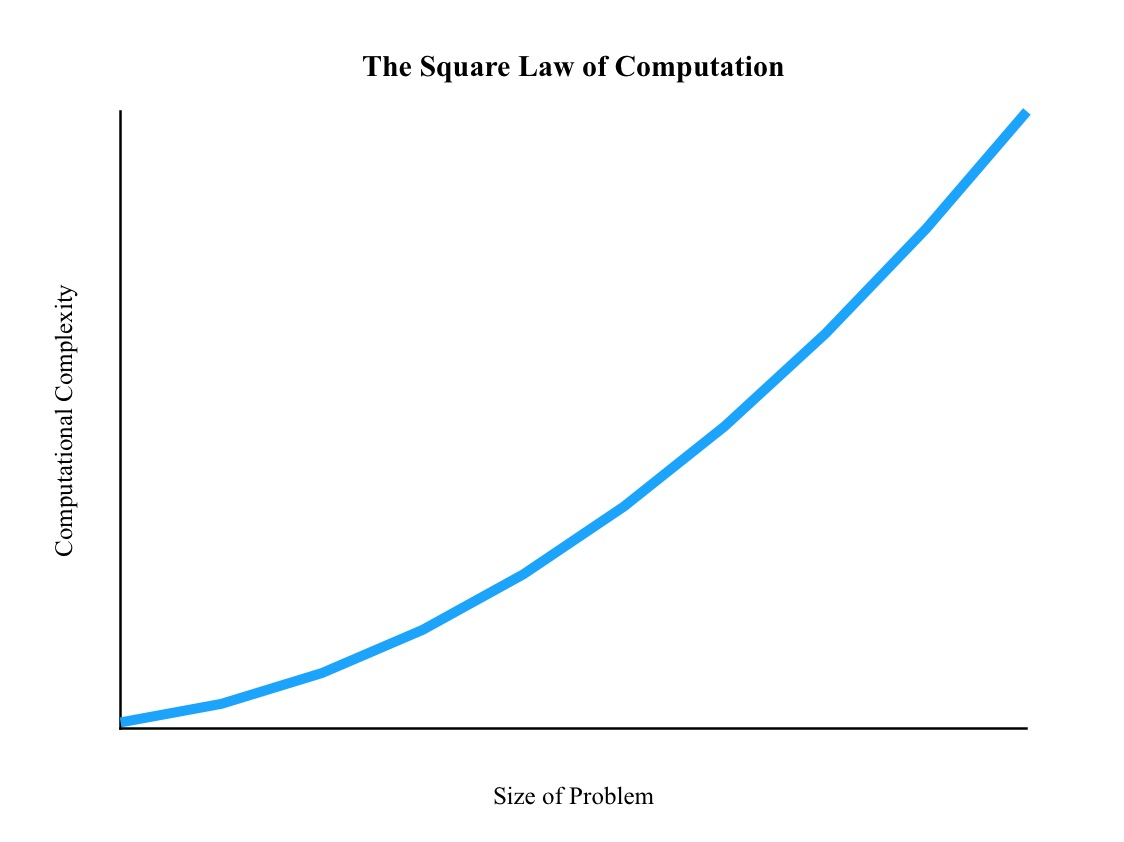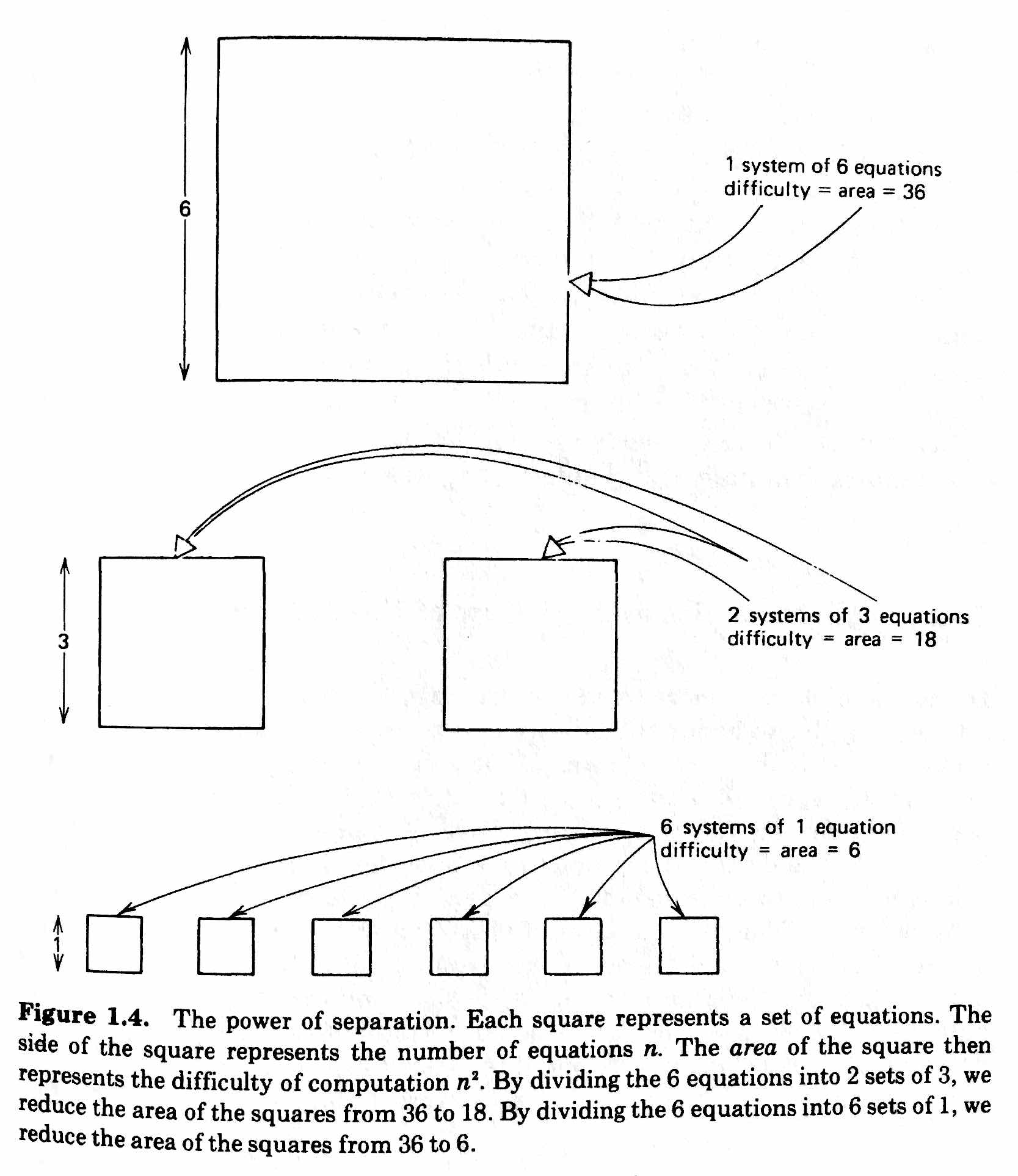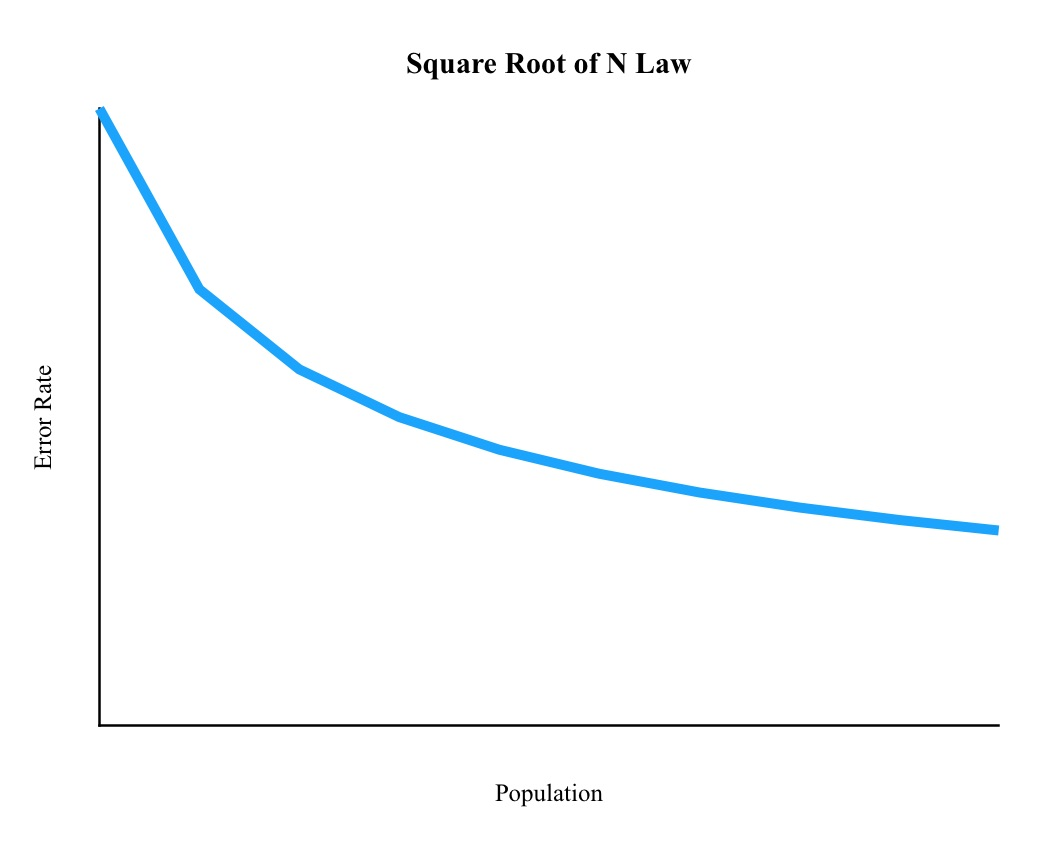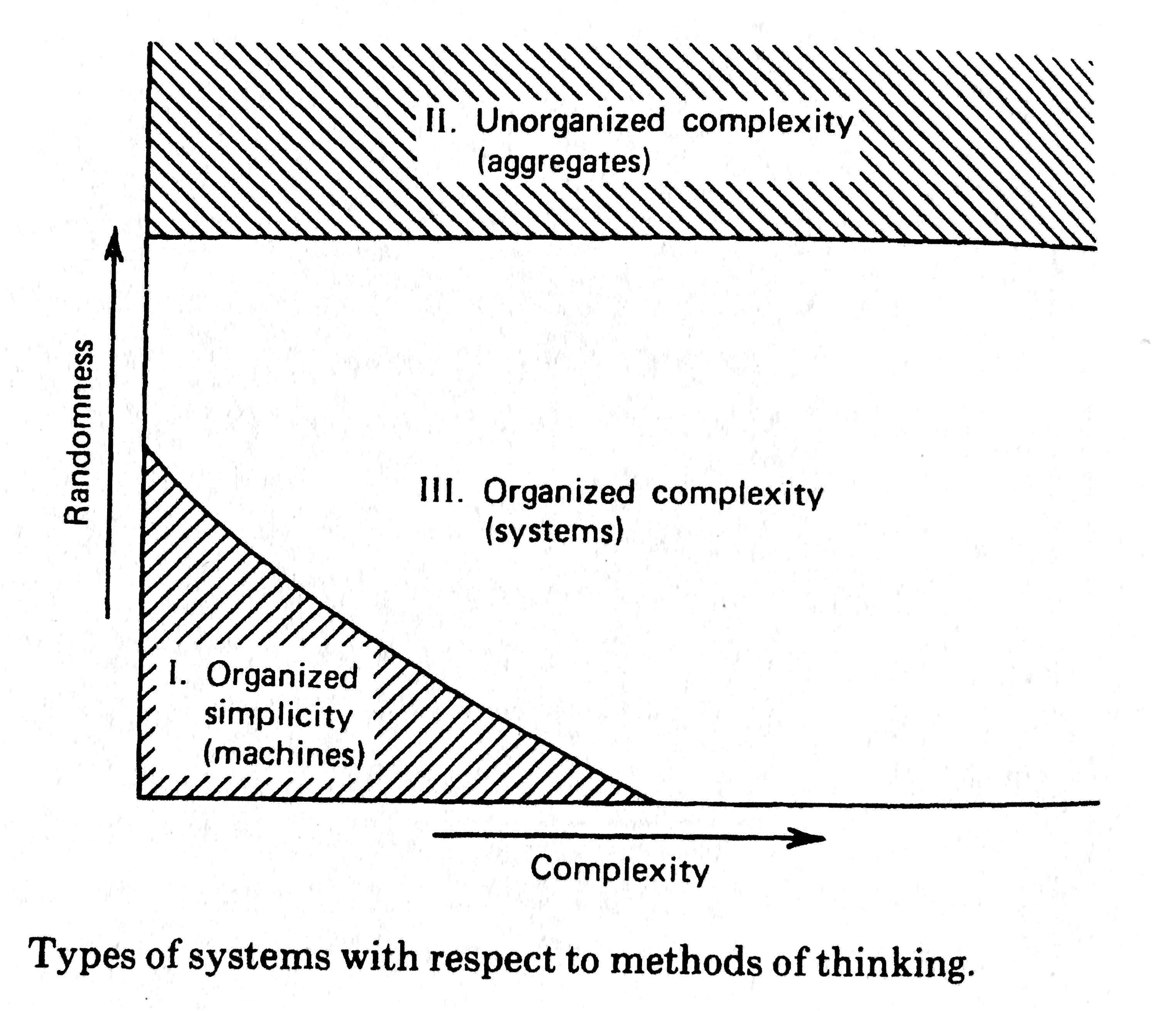# Book Notes – An Introduction to General Systems Thinking## Chapter 1: The Problem

The first step to knowledge is the confession of ignorance.

The question “How much time and money?” lies at the very foundation of the general systems movement.

We do not need exact measures. Instead, we merely want to estimate how the amount of computation increases as the size of the problem increases.

Unless some simplifications can be made, the amount of computation involved increases at least as fast as the square of the number of equations.

The Square Law of Computation: n2• Isolated Behaviour: how each object behaves by itself
• Interaction: how the behaviour of each body affects that of the other
• Field: how things will behave if neither of the bodies is present

### Simplification

A general systems thinker’s task is to understand the simplifying assumptions of a science.

objects of interest and well defined conditions that delimit its domain of application and magnify the power of prediction.

A separation of a system into noninteracting subsystems is an extremely important technique known to all developed sciences.The Law of Large Numbers: The larger the population, the more likely we are to observe values that are close to the predicted average values.

An organism must have a comparatively gross structure in order to enjoy the benefit of fairly accurate laws.The Law of Medium Numbers: Murphy’s Law: Anything that can happen, will happen.• Randomnes ~ Diversity
• Complexity ~ Population
• High randomness + high complexity: Use aggregates
• Low randomness + low complexity: Use mechanics
• Medium randomness + Medium complexity: Use Systems Thinking